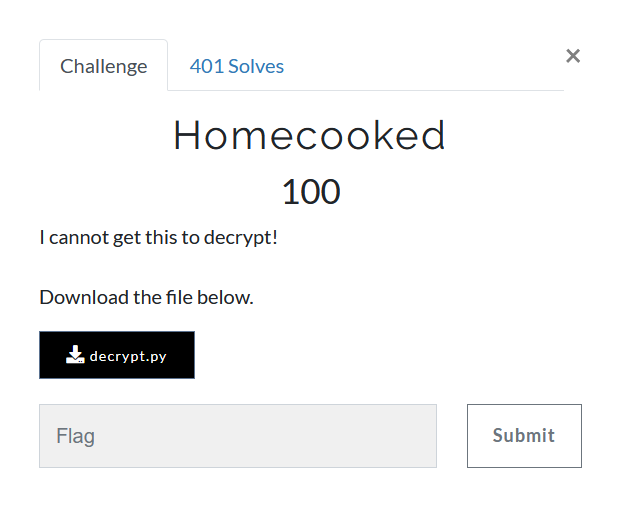# HomecookedWe are provided decrypt.py, the contents of which read

import base64
num = 0
count = 0
cipher_b64 = b"MTAwLDExMSwxMDAsOTYsMTEyLDIxLDIwOSwxNjYsMjE2LDE0MCwzMzAsMzE4LDMyMSw3MDIyMSw3MDQxNCw3MDU0NCw3MTQxNCw3MTgxMCw3MjIxMSw3MjgyNyw3MzAwMCw3MzMxOSw3MzcyMiw3NDA4OCw3NDY0Myw3NTU0MiwxMDAyOTAzLDEwMDgwOTQsMTAyMjA4OSwxMDI4MTA0LDEwMzUzMzcsMTA0MzQ0OCwxMDU1NTg3LDEwNjI1NDEsMTA2NTcxNSwxMDc0NzQ5LDEwODI4NDQsMTA4NTY5NiwxMDkyOTY2LDEwOTQwMDA="

def a(num):
if (num > 1):
for i in range(2,num):
if (num % i) == 0:
return False
break
return True
else:
return False

def b(num):
my_str = str(num)
rev_str = reversed(my_str)
if list(my_str) == list(rev_str):
return True
else:
return False

cipher = base64.b64decode(cipher_b64).decode().split(",")

while(count < len(cipher)):
if (a(num)):
if (b(num)):
print(chr(int(cipher[count]) ^ num), end='', flush=True)
count += 1
if (count == 13):
num = 50000
if (count == 26):
num = 500000
else:
pass
num+=1

print()


Here two functions a and b are of primary focus,

def a(num):
if (num > 1):
for i in range(2,num):  #From all numbers from 2 to num - 1
if (num % i) == 0:  #check if the number i divides num
return False    #if it divides, then its composite
break
return True  # No such number found hence prime
else:
return False


Seems like, its looping over all possible numbers to check if the provided number num is a prime or not

def b(num):
my_str = str(num) # string representation of number
rev_str = reversed(my_str)  # reverse the string
if list(my_str) == list(rev_str): # check if both equal
return True  # the input is a palindrome
else:
return False


This function just checks if num is a palindrome

The cipher_b64 is simply a base64 encoding of a comma separated list of numbers

100,111,100,96,112,21,209,166,216,140,330,318,321,70221,70414,70544,71414,71810,72211,72827,73000,73319,73722,74088,74643,75542,1002903,1008094,1022089,1028104,1035337,1043448,1055587,1062541,1065715,1074749,1082844,1085696,1092966,1094000


It is simply taking number from the list and xoring with next prime-palindrome to give the character in sets of 13. Lets simply run the decrypt.py to see what happens

python3 decrypt.py
flag{pR1m3s_4re_co0ler_Wh3


Hmm, seems stuck. What’s the issue?
The issue is with function a which checks for prime by dividing it by all numbers.
This can be done pretty efficiently by better primality tests.
One could simply use is_prime from gmpy2 library of python and replace the function a

import base64
import gmpy2
num = 0
count = 0
cipher_b64 = b"MTAwLDExMSwxMDAsOTYsMTEyLDIxLDIwOSwxNjYsMjE2LDE0MCwzMzAsMzE4LDMyMSw3MDIyMSw3MDQxNCw3MDU0NCw3MTQxNCw3MTgxMCw3MjIxMSw3MjgyNyw3MzAwMCw3MzMxOSw3MzcyMiw3NDA4OCw3NDY0Myw3NTU0MiwxMDAyOTAzLDEwMDgwOTQsMTAyMjA4OSwxMDI4MTA0LDEwMzUzMzcsMTA0MzQ0OCwxMDU1NTg3LDEwNjI1NDEsMTA2NTcxNSwxMDc0NzQ5LDEwODI4NDQsMTA4NTY5NiwxMDkyOTY2LDEwOTQwMDA="

def a(num):
return gmpy2.is_prime(num)

def b(num):
return str(num) == str(num)[::-1]

cipher = base64.b64decode(cipher_b64).decode().split(",")

while(count < len(cipher)):
if (a(num)):
if (b(num)):
print(chr(int(cipher[count]) ^ num), end='', flush=True)
count += 1
if (count == 13):
num = 50000
if (count == 26):
num = 500000
else:
pass
num+=1

print()


Now running solve.py spews the flag instantly

### flag{pR1m3s_4re_co0ler_Wh3n_pal1nDr0miC}

jekyll.environment != "beta" -%}